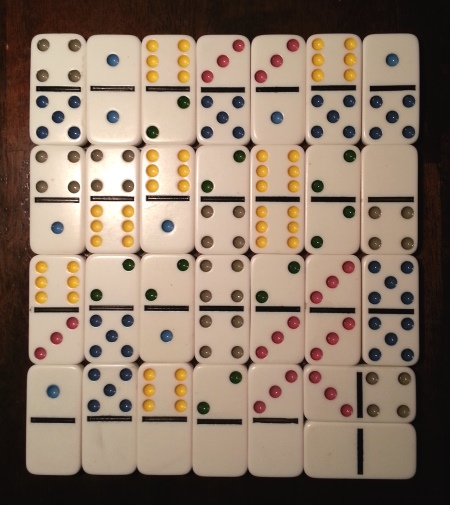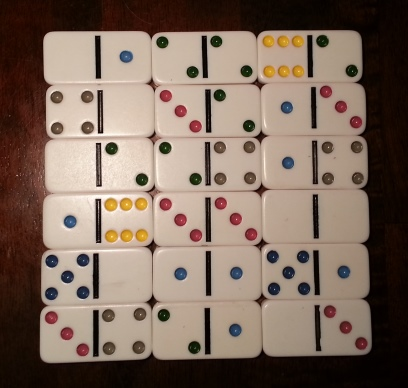# Magic square made of dominoes

You can arrange a standard set of dominoes into a magic square of sorts. There are 28 dominoes, each with two ends, so the number of ends isn’t a perfect square. But if you ignore the row of blanks at the bottom, you have a 7 by 7 square where every row, column, and diagonal add to 24. (Technically this isn’t a magic square since some numbers repeat.)If you don’t use all the dominoes you can make an actual square, no extra row to ignore:I found the design of these magic squares in The Zen of Magic Squares, Circles, and Stars by Cliff Pickover. He credits Mathematical recreations and essays by Ball and Coxeter.

While the most common set of dominoes goes up to double six, there are other possibilities. I made the images above by pulling out the double six subset out of a set of double nine dominoes. Is it possible to make magic squares like the first photo above out of a full set of dominoes up to double n for other values of n? Not if n is odd, but perhaps if n is even.

You can at least arrange the dominoes into a square. A set of double n dominoes has (n+1)(n + 2)/2 pieces, so (n+1)(n + 2) squares. With a row of n + 1 blanks on the bottom, this leaves (n + 1)2 squares in the magic square. The total number of points in a set of double n dominoes is n(n + 1)(n + 2)/2, so each row, column, and diagonal would have to sum to n(n + 2)/2. This means you cannot arrange a set of double n dominoes into a magic square if n is odd. So, for example, it won’t work for double nine dominoes. Is it possible when n is even? Certainly if n = 6, but what about other values of n?

## One thought on “Magic square made of dominoes”

1. Try N = 2. quick calc says it will not work.